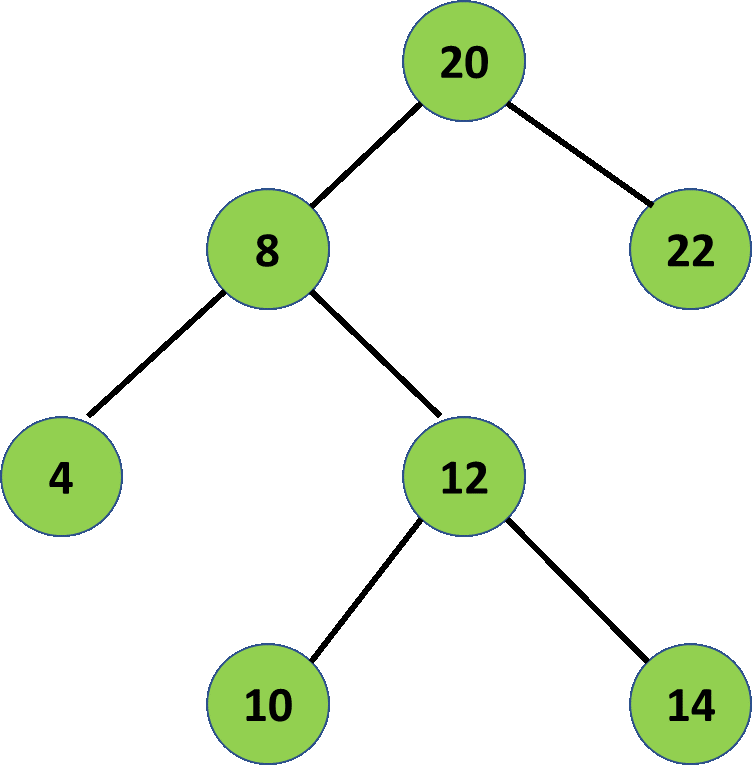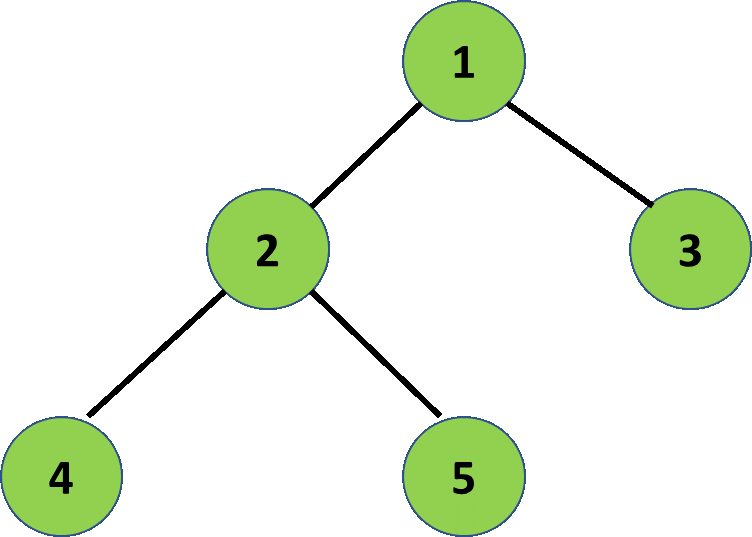Related Articles
Print the nodes that are just above the leaf node
• Last Updated : 11 Nov, 2020

Given a binary tree consisting of N nodes, the task is to print the nodes that are just above the leaf node.
Examples:

Input: N = 7, Below is the given Binary Tree:Output: 20 8 12
Explanation:
Node 20 is just above the leaf node 22.
Node 8 is just above the leaf node 4.
Node 12 is just above the leaf nodes 10 and 14.
Input: N = 5, Below is the given Binary Tree:Output: 1 2
Explanation:
Node 1 is just above the leaf node 3.
Node 2 is just above the leaf nodes 4 and 5.

Approach: The idea is to traverse the tree and for each node, check if it can be the one which is just above the leaf node. For that, the current node must have children and at least one of the children should be a leaf node. Below are the steps:

• Traverse the tree And check each node.
• If the current node has two children, check if any of them is a root node. If yes, then print the current node.
• If the current node has only a left or right child, then check if that left or the right child is a leaf node. If yes, then print the current node.
• Else, continue traversing the tree and move to the next node.

Below is the implementation of the above approach:

## C++14

 `// C++ program for the above approach` `#include ` `using` `namespace` `std;`   `// Node of tree` `struct` `node {` `    ``int` `data;` `    ``struct` `node *left, *right;` `};`   `// Creates and initializes a new` `// node for the tree` `struct` `node* newnode(``int` `data)` `{` `    ``struct` `node* temp = ``new` `node();` `    ``temp->data = data;` `    ``temp->left = temp->right = NULL;` `    ``return` `temp;` `}`   `// Prints all nodes which are just` `// above leaf node` `void` `cal(``struct` `node* root)` `{`   `    ``// If tree is empty` `    ``if` `(root == NULL) {` `        ``return``;` `    ``}`   `    ``// If it is a leaf node` `    ``if` `(root->left == NULL` `        ``&& root->right == NULL) {` `        ``return``;` `    ``}`   `    ``// For internal nodes` `    ``else` `{`   `        ``// If node has two children` `        ``if` `(root->left != NULL` `            ``&& root->right != NULL) {`   `            ``if` `((root->left->left == NULL` `                 ``&& root->left->right == NULL)` `                ``|| (root->right->left == NULL` `                    ``&& root->right->right == NULL)) {`   `                ``cout << root->data << ``" "``;` `            ``}` `        ``}`   `        ``// If node has only left child` `        ``if` `(root->left != NULL` `            ``&& root->right == NULL) {`   `            ``if` `(root->left->left == NULL` `                ``&& root->left->right == NULL) {`   `                ``cout << root->data << ``" "``;` `            ``}` `        ``}`   `        ``// If node has only right child` `        ``if` `(root->right != NULL` `            ``&& root->left == NULL) {`   `            ``if` `(root->right->left == NULL` `                ``&& root->right->right == NULL) {`   `                ``cout << root->data << ``" "``;` `            ``}` `        ``}` `    ``}`   `    ``// Recursively Call for left` `    ``// and right subtree` `    ``cal(root->left);` `    ``cal(root->right);` `}`   `// Driver Code` `int` `main()` `{` `    ``// Construct a tree` `    ``node* root = newnode(20);` `    ``root->left = newnode(8);` `    ``root->right = newnode(22);` `    ``root->left->left = newnode(4);` `    ``root->left->right = newnode(12);` `    ``root->left->right->left = newnode(10);` `    ``root->left->right->right = newnode(14);`   `    ``// Function Call` `    ``cal(root);`   `    ``return` `0;` `}`

## Java

 `// Java program for the above approach` `import` `java.util.*;`   `// Class containing the left and right ` `// child of current node and the ` `// key value ` `class` `Node ` `{ ` `    ``int` `data; ` `    ``Node left, right; `   `    ``// Constructor of the class ` `    ``public` `Node(``int` `item) ` `    ``{ ` `        ``data = item; ` `        ``left = right = ``null``; ` `    ``} ` `} `   `class` `GFG{` `    `  `Node root; `   `// Prints all nodes which are just ` `// above leaf node ` `static` `void` `cal(Node root) ` `{ `   `    ``// If tree is empty ` `    ``if` `(root == ``null``)` `    ``{ ` `        ``return``; ` `    ``} `   `    ``// If it is a leaf node ` `    ``if` `(root.left == ``null` `&& ` `       ``root.right == ``null``)` `    ``{ ` `        ``return``; ` `    ``} `   `    ``// For internal nodes ` `    ``else` `    ``{ `   `        ``// If node has two children ` `        ``if` `(root.left != ``null` `&& ` `           ``root.right != ``null``)` `        ``{ ` `            ``if` `((root.left.left == ``null` `&&` `                ``root.left.right == ``null``) ||` `               ``(root.right.left == ``null` `&& ` `               ``root.right.right == ``null``)) ` `            ``{ ` `                ``System.out.print(root.data + ``" "``); ` `            ``} ` `        ``} `   `        ``// If node has only left child ` `        ``if` `(root.left != ``null` `&& ` `           ``root.right == ``null``) ` `        ``{ ` `            ``if` `(root.left.left == ``null` `&&` `               ``root.left.right == ``null``) ` `            ``{ ` `                ``System.out.print(root.data + ``" "``); ` `            ``} ` `        ``} `   `        ``// If node has only right child ` `        ``if` `(root.right != ``null` `&& ` `             ``root.left == ``null``)` `        ``{ ` `            ``if` `(root.right.left == ``null` `&&` `               ``root.right.right == ``null``) ` `            ``{ ` `                ``System.out.print(root.data + ``" "``); ` `            ``} ` `        ``} ` `    ``} `   `    ``// Recursively call for left ` `    ``// and right subtree ` `    ``cal(root.left); ` `    ``cal(root.right); ` `}` `    `  `// Driver Code` `public` `static` `void` `main (String[] args) ` `{` `    ``GFG tree = ``new` `GFG(); `   `    ``tree.root = ``new` `Node(``20``); ` `    ``tree.root.left = ``new` `Node(``8``); ` `    ``tree.root.right = ``new` `Node(``22``); `   `    ``tree.root.left.left = ``new` `Node(``4``); ` `    ``tree.root.left.right = ``new` `Node(``12``); `   `    ``tree.root.left.right.left = ``new` `Node(``10``);` `    ``tree.root.left.right.right = ``new` `Node(``14``);` `    `  `    ``// Function call ` `    ``cal(tree.root); ` `}` `}`   `// This code is contributed by offbeat`

## Python3

 `# Python3 program for the` `# above approach`   `# Node of tree` `class` `newNode:` `  `  `    ``def` `__init__(``self``, data):` `      `  `        ``self``.data ``=` `data` `        ``self``.left ``=` `None` `        ``self``.right ``=` `None`   `# Creates and initializes a new` `# node for the tree`   `# Prints all nodes which are ` `# just above leaf node` `def` `cal(root):` `  `  `    ``# If tree is empty` `    ``if` `(root ``=``=` `None``):` `        ``return`   `    ``# If it is a leaf node` `    ``if` `(root.left ``=``=` `None` `and` `        ``root.right ``=``=` `None``):` `        ``return`   `    ``# For internal nodes` `    ``else``:` `      `  `        ``# If node has two children` `        ``if` `(root.left !``=` `None` `and` `            ``root.right !``=` `None``):` `            ``if` `((root.left.left ``=``=` `None` `and` `                 ``root.left.right ``=``=` `None``) ``or` `                ``(root.right.left ``=``=` `None` `and` `                 ``root.right.right ``=``=` `None``)):` `                ``print``(root.data, end ``=` `" "``)`   `        ``# If node has only left child` `        ``if` `(root.left !``=` `None` `and` `            ``root.right ``=``=` `None``):` `            ``if` `(root.left.left ``=``=` `None` `and` `                ``root.left.right ``=``=` `None``):` `                ``print``(root.data, end ``=` `" "``)`   `        ``# If node has only right child` `        ``if` `(root.right !``=` `None` `and` `            ``root.left ``=``=` `None``):` `            ``if` `(root.right.left ``=``=` `None` `and` `                ``root.right.right ``=``=` `None``):` `                ``print``(root.data, end ``=` `" "``) `   `    ``# Recursively Call for left` `    ``# and right subtree` `    ``cal(root.left)` `    ``cal(root.right)`   `# Driver Code` `if` `__name__ ``=``=` `'__main__'``:` `  `  `    ``# Construct a tree` `    ``root ``=` `newNode(``20``)` `    ``root.left ``=` `newNode(``8``)` `    ``root.right ``=` `newNode(``22``)` `    ``root.left.left ``=` `newNode(``4``)` `    ``root.left.right ``=` `newNode(``12``)` `    ``root.left.right.left ``=` `newNode(``10``)` `    ``root.left.right.right ``=` `newNode(``14``)`   `    ``# Function Call` `    ``cal(root)`   `# This code is contributed by SURENDRA_GANGWAR`

## C#

 `// C# program for the above approach` `using` `System;`   `// Class containing the left and right ` `// child of current node and the ` `// key value ` `class` `Node ` `{ ` `    ``public` `int` `data; ` `    ``public` `Node left, right; `   `    ``// Constructor of the class ` `    ``public` `Node(``int` `item) ` `    ``{ ` `        ``data = item; ` `        ``left = right = ``null``; ` `    ``} ` `} `   `class` `GFG{    ` `Node root; `   `// Prints all nodes which are just ` `// above leaf node ` `static` `void` `cal(Node root) ` `{ ` `    ``// If tree is empty ` `    ``if` `(root == ``null``)` `    ``{ ` `        ``return``; ` `    ``} `   `    ``// If it is a leaf node ` `    ``if` `(root.left == ``null` `&& ` `        ``root.right == ``null``)` `    ``{ ` `        ``return``; ` `    ``} `   `    ``// For internal nodes ` `    ``else` `    ``{ ` `        ``// If node has two children ` `        ``if` `(root.left != ``null` `&& ` `            ``root.right != ``null``)` `        ``{ ` `            ``if` `((root.left.left == ``null` `&&` `                 ``root.left.right == ``null``) ||` `                ``(root.right.left == ``null` `&& ` `                 ``root.right.right == ``null``)) ` `            ``{ ` `                ``Console.Write(root.data + ``" "``); ` `            ``} ` `        ``} `   `        ``// If node has only left child ` `        ``if` `(root.left != ``null` `&& ` `            ``root.right == ``null``) ` `        ``{ ` `            ``if` `(root.left.left == ``null` `&&` `                ``root.left.right == ``null``) ` `            ``{ ` `                ``Console.Write(root.data + ``" "``); ` `            ``} ` `        ``} `   `        ``// If node has only right child ` `        ``if` `(root.right != ``null` `&& ` `            ``root.left == ``null``)` `        ``{ ` `            ``if` `(root.right.left == ``null` `&&` `                ``root.right.right == ``null``) ` `            ``{ ` `                ``Console.Write(root.data + ``" "``); ` `            ``} ` `        ``} ` `    ``} `   `    ``// Recursively call for left ` `    ``// and right subtree ` `    ``cal(root.left); ` `    ``cal(root.right); ` `}` `    `  `// Driver Code` `public` `static` `void` `Main(String[] args) ` `{` `    ``GFG tree = ``new` `GFG(); ` `    ``tree.root = ``new` `Node(20); ` `    ``tree.root.left = ``new` `Node(8); ` `    ``tree.root.right = ``new` `Node(22); ` `    ``tree.root.left.left = ``new` `Node(4); ` `    ``tree.root.left.right = ``new` `Node(12); ` `    ``tree.root.left.right.left = ``new` `Node(10);` `    ``tree.root.left.right.right = ``new` `Node(14);` `    `  `    ``// Function call ` `    ``cal(tree.root); ` `}` `}`   `// This code is contributed by Rajput-Ji`

Output:

```20 8 12

```

Time Complexity: O(N)
Auxiliary Space: O(1)My Personal Notes arrow_drop_up
Recommended Articles
Page :